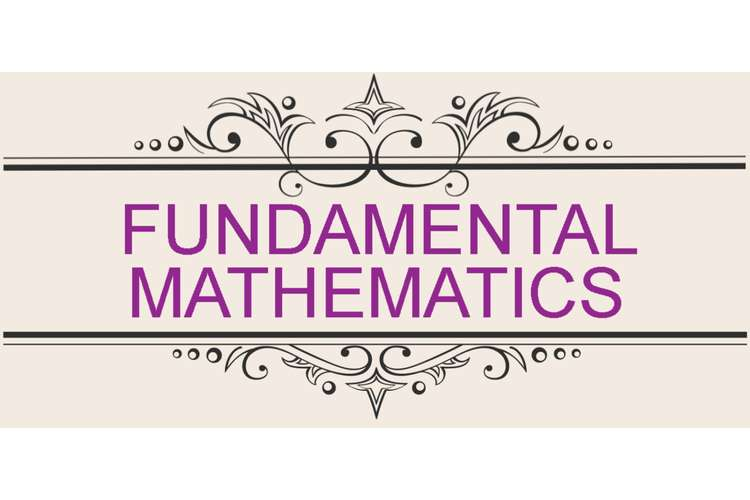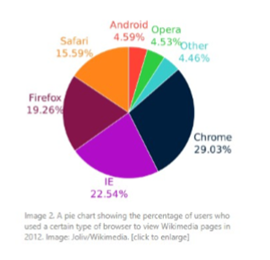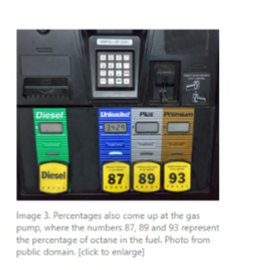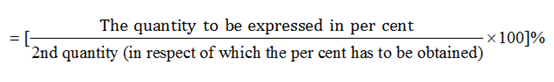# Fundamental Mathematics Paper Editing Services### This article discusses the basics of percentage system of mathematics and its day to day application in life.  Mathematically percentage is a part of a whole expressed as a part of 100. Basically, it is the business method of representation of the proportion of full.

This article contains the following two images:Figure 1: image 1                                                                     Figure 2 : image 2

These are the images used here in the article to completely justify the definitions and to represent the definition in picture form.

### Figure 1:

As the description in the image shows that it is a pie chart that is used here to represent the percentage of users who used a certain type of browser to view Wikimedia images in the year 2012.

Per cant is a Latin word in which per means ‘by’ and cent means ‘100’ Percentage is generally the fraction with 100 in the denominator. It is represented by using the percentage itself, like 30 percent, 100 percent, etc. It is often represented by using the symbol ‘%’. The percentage is like the most important part of the implementation of mathematical tools. The pie chart here is totally connected with text in the article.

Various concepts of mathematics like percentage, ratio, and fractions are used to correlate with the functioning of percentage.

### Percentage:

Percentimplies “for every hundred” and the sign % is read as a percentage and x % is read as x percent. In other words, a fraction with denominator 100 is called a percent. For example, 20 % means 20/100 (i.e. 20 parts from 100). This can also be written as 0.2.

Percentrefers to “for every 100” and the symbol used to represent is ‘%’. In simple ways, a number with the denominator as 100 is called as a percentage. For instance, 52% is equal to 52/100 which means 52 parts of 100. This can also be represented as 0.52.

### Representing a quantity aspercent(%) in comparison with other quantity:The above-shown formula is used to represent any quantity with respect to other quantity. E.g.:

Calculate the percentage of 60 in 240?

Answer:First we will rewrite the given no. in fraction form:
60/240 = ¼
Multiplying the num. and denominatorwith 25 to make the denominator 100
(1×25)/(4×25) = 25/100= 25%

The percentage can be displayed using many methods like 50 percent, 50%, or 50/100 or can also be written as 0.5.

There isa method of conversion of decimal to a percent:

Move the decimal in right up to 2 places and place the %sign at the end. E.g. 0.79 means 79%.

Percent to a fraction:

a%= a/100 for e.g. 30%=30/100

The image 1 used in the article is the perfect representation of percentage. The pie chart shows the browser user percentage for viewing Wikimedia image. Following qualitative data can be retrieved from the pie chart:

Android -4.59%Safari-15.59

Chrome- 29.03%Opera -4.53%

IE-22.54%Firefox-19.26%

This reveals the fact about the use of different web browsers to view any image. The circle of the pie, chart represents the whole or the 100%  user, i.e. the total user who want to view the Wikimedia image. The different percentages mentioned with the web browser names show the percent or proportion of a particular browser use. This is totally relevant to the text as the article also discusses the concept of percentage and its implementation or application in day to day life.

### Figure 2:

It displays the day to day application of the percentage. It represents the percentage of octane inside the fuel at the fuel pump.

### Percentages find a variety of application in day to day life:

1. The amount of cholesterol in any food is written over the packaging of the food products in the form of percentage to show the healthiness of the food.
2. The content of chemical inside different cleaning liquids and soaps are displayed by using per cent.
3. Medicines also come up with a detailed content description over the packing of medicine.
4. The offer of different products or services is displayed by using % off on the price. E.g. flat 40% off on product pricing means the product can be bought on price 40% less than the original price.
5. In the stock market, the percent is used to display the amount of profit or less made by any stock.

Apart from percent ratio and proportions are also used to display the relationship management between different quantities. Ratio helps in comparing two quantities. The ratio is just like percent but it is between the number of same kinds. Two different quantities cannot be compared using ratio. It basically divides two quantities of the same kind.

Denoted by a÷ b/ a:b and read as a ratio b. since ration is the comparison between two equal quantity it has no unit. It represents that how much a quantity is greater than other quantity. For instance: the ratio of men: women in the office are 1:2 that means the no of women in the officeis half in no to no of men in office.

So basically both the images used here are very much relevant to the text here as they both provide the best idea of the topic discussed inside the article by displaying the use of percentage in the day to day life and by displaying the part of a whole with respect to some content.